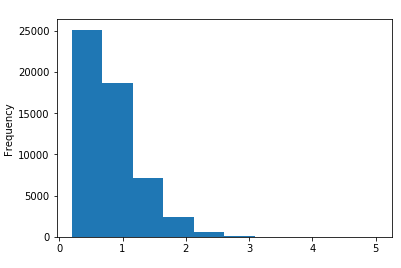﻿ Pandas Practice Set-1: Create a histogram of the 'carat' Series of diamonds DataFrame - w3resource# Pandas Practice Set-1: Create a histogram of the 'carat' Series of diamonds DataFrame

## Pandas Practice Set-1: Exercise-37 with Solution

Write a Pandas program to create a histogram of the 'carat' Series (distribution of a numerical variable) of diamonds DataFrame.

Sample Solution:

Python Code:

``````import pandas as pd
print("Original Dataframe:")
print("\nHistogram of the 'carat' Series (distribution of a numerical variable) of diamonds DataFrame.")
diamonds.carat.plot(kind='hist')
``````

Sample Output:

```Original Dataframe:
carat      cut color clarity  depth  table  price     x     y     z
0   0.23    Ideal     E     SI2   61.5   55.0    326  3.95  3.98  2.43
1   0.21  Premium     E     SI1   59.8   61.0    326  3.89  3.84  2.31
2   0.23     Good     E     VS1   56.9   65.0    327  4.05  4.07  2.31
3   0.29  Premium     I     VS2   62.4   58.0    334  4.20  4.23  2.63
4   0.31     Good     J     SI2   63.3   58.0    335  4.34  4.35  2.75

Histogram of the 'carat' Series (distribution of a numerical variable) of diamonds DataFrame.```

Python Code Editor:

Have another way to solve this solution? Contribute your code (and comments) through Disqus.

What is the difficulty level of this exercise?

﻿

## Python: Tips of the Day

Python: Unzipping

```name = 'abcdef'
suffix = [1,2,3,4,5,6]
result = zip(name, suffix)
--> returns (a,1),(b,2),(c,3),(d,4),(e,5),(f,6)
unzipped = zip(*result)
```